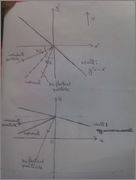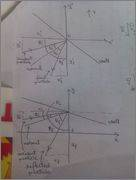# Lorentz tranformation of law of reflection

• spaghetti3451
In summary, the wall has a gentler slope and the incident angle decreases and the reflected angle increases with the increase of the velocity of the frame ##S'##.

## Homework Statement

Consider an inertial frame ##S## with coordinates ##x^{\mu}=(t,x,y,z)##, and a frame ##S'## with coordinates ##x^{\mu'}## related to ##S## by a boost with velocity parameter ##v## along the ##y##-axis. Imagine we have a wall at rest in ##S'##, lying along the line ##x'=-y'##. From the point of view of ##S##, what is the relationship between the incident angle of a ball hitting the wall (travelling in the ##x##-##y## plane) and the reflected angle? What about the velocity before and after?

## The Attempt at a Solution

From the point of view of ##S'##, the incident angle equals the reflected angle.

The frame ##S'## travels parallel to the ##y##-axis of the frame ##S##. Therefore, ##y'=-x' \implies \gamma\ y = - x##.

Therefore, from the point of view of the ##S## frame, the wall has a gentler slope (i.e., slope of smaller magnitude).

Will the trajectories also change their directions in the ##S## frame?

The fact that the wall has a gentler slope in the ##S## frame is a true fact. However, I think that it is not of much use in solving the problem. It can, however, help in developing a feel for how the wall and the trajectories of the incident and the reflected particles appear from the ##S## frame. In particular, I think that, in the ##S## frame, (due to Lorentz contraction only along the ##y##-direction, and no Lorentz contraction along the ##x##- direction) the incident angle decreases and the reflected angle increases (with respect to the Lorentz-transformed normal to the wall) with the increase of the velocity of the ##S'## frame. This is illustrated in the figure below.My additional progress with the problem so far:Using the figure above,

##1 = \text{tan}\ 45^{\circ} = \text{tan}\ \phi'_{i}=\frac{Y'_{i}}{X'_{i}}=\frac{\gamma\ Y_{i}}{X_{i}}=\gamma\ \text{tan}\ \phi_{i}## so that ##\text{tan}\ \phi_{i}=\frac{1}{\gamma}##

and

##1 = \text{tan}\ 45^{\circ} = \text{tan}\ \phi'_{f}=\frac{X'_{f}}{Y'_{f}}=\frac{X_{f}}{\gamma\ Y_{f}}=\frac{1}{\gamma}\ \text{tan}\ \phi_{f}## so that ##\text{tan}\ \phi_{f}=\gamma##.Furthermore, using the figure above,

##\text{tan}\ (\phi'_{i}-\theta'_{i})=\frac{y'_{i}}{x'_{i}}=\frac{\gamma\ y_{i}}{x_{i}}=\gamma\ \text{tan}\ (\phi_{i}-\theta_{i})##

and

##\text{tan}\ (\phi'_{f}-\theta'_{f})=\frac{x'_{f}}{y'_{f}}=\frac{x_{f}}{\gamma\ y_{f}}=\frac{1}{\gamma}\ \text{tan}\ (\phi_{f}-\theta_{f})##

so that

##\text{tan}\ (\phi'_{i}-\theta'_{i})=\text{tan}\ (\phi'_{f}-\theta'_{f})##

##\implies \gamma\ \text{tan}\ (\phi_{i}-\theta_{i}) = \frac{1}{\gamma}\ \text{tan}\ (\phi_{f}-\theta_{f})##

##\implies \gamma^{2}\ \frac{\text{tan}\ \phi_{i}-\text{tan}\ \theta_{i}}{1+\text{tan}\ \phi_{i}\text{tan}\ \theta_{i}}=\frac{\text{tan}\ \phi_{f}-\text{tan}\ \theta_{f}}{1+\text{tan}\ \phi_{f}\text{tan}\ \theta_{f}}##

##\implies \gamma^{2}\ \frac{\frac{1}{\gamma} - \text{tan}\ \theta_{i}}{1+\frac{1}{\gamma}\text{tan}\ \theta_{i}}=\frac{\gamma-\text{tan}\ \theta_{f}}{1+\gamma\ \text{tan}\ \theta_{f}}##

##\implies \gamma^{2}\ \frac{1- \gamma\ \text{tan}\ \theta_{i}}{\gamma +\text{tan}\ \theta_{i}}=\frac{\gamma-\text{tan}\ \theta_{f}}{1+\gamma\ \text{tan}\ \theta_{f}}##

Am I doing ok? Am I heavy weather out of a simple problem?